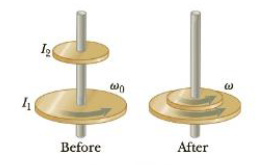Chapter 8, Problem 73P

Chapter
Section
Textbook Problem

A cylinder with moment of inertia I1 rotates with angular velocity ω0 about a frictionless vertical axle. A second cylinder, with moment of inertia I2, initially not. rotating, drops onto the first cylinder (Fig. P8.73). Because the surfaces are rough, the two cylinders eventually reach the same angular speed ω. (a) Calculate ω. (b) Show that kinetic energy is lost in this situation, and calculate the ratio of the final lo the initial kinetic energy.Figure P8.73

(a)

To determine
The angular speed ω of both cylinders.

Explanation

The conservation of angular momentum Li=Lf(I1+I2)ω=I0ω0 is used for the calculation of angular speed of the disks. The angular speed of the first cylinder is ω0 , angular speed of the second cylinder before its drop on the first one is 0rad/s , the final angular speed of both cylinders after the drop of second on the first one is ω , moment of inertia of first cylinder before the drop of second cylinder is I1 , the moment of inertia of second cylinder before its drop is

(b)

To determine
The kinetic energy lost in the situation and the ratio of final to initial kinetic energy.

Still sussing out bartleby?

Check out a sample textbook solution.

See a sample solution

The Solution to Your Study Problems

Bartleby provides explanations to thousands of textbook problems written by our experts, many with advanced degrees!

Get Started